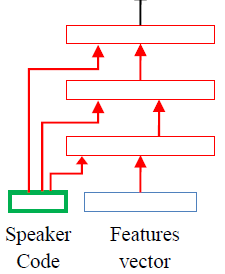# How to implement the follow structure of network

Hi, I wantto implement the following network, each layer receives actication of the lower layer along with speaker code, and using back-propagation to update the speaker code. but I don’t know how to implement it.In your desired architecture the inputs of the layers are a combination of speaker code and feature previous layer outputs or the feature vector input. I guess you want to condition on the speaker code.

You could pass the speaker code as additional input, e.g. by concatenating it to the regular layer input. Another very simple method is to use addition to mix in the speaker code. From your diagram I cannot see what kind of network you want, e.g. if the red layers are fully-connected layers or conv layers. The first thing you could do is to write down all the tensor dimensionalities and then think about bringing them into a ‘compatible form’, e.g. if the dimensionality does not match you could try use a linear layer to adapt it.

I tried to implement this structure, but I‘am not sure that’s right. The following code just a example.

``````class TestDNN(nn.Module):
def __init__(self):
super(TestDNN, self).__init__()
self.speaker_code = nn.Parameter(torch.randn(1))
self.fc1 = nn.Linear(2, 2, bias=False)
self.fc2 = nn.Linear(3, 1, bias=False)

def forward(self, input):
x = torch.cat((self.speaker_code.repeat(input.shape,1), input), 1)
x = self.fc1(x)
x = torch.cat((self.speaker_code.repeat(input.shape,1), x),1)
return self.fc2(x)
``````